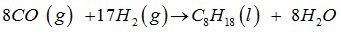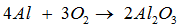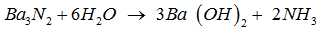# Topic: Some Basic Concepts of Chemistry (Test 1)

Topic: Some Basic Concepts of Chemistry
Q.1
Chemistry does not play a major role in
A. Explaining ozone depletion
B. Design and synthesis new materials having specific magnetic, electric and optical properties.
C. Explaining superconductivity
D. Large scale production of a variety of fertilizers
Explaination / Solution:

Chemistry does not deal in explaining superconductivity.

*In superconducting materials the charecteristics of superconductivity appear when the temperature is lowered below a critical temperature.

*The onset of superconductivity is accompanied  by abrupt changes in physical properties which are more related to phase transitions of the material .

These aspects of  studies in properties of materials  are better related to studies in the fields of Physics , eventhough  principles involved in Chemistry & Physics go hand to hand.

Workspace
Report
Q.2

Consider the chemical  equation ,

----- KOH + ----- H3PO4 → ----- K3PO4 + ----- H2O.

The equation can be balanced by inserting the following in blank spaces

A. 3,2,1,2
B. 6.1.2.3
C. 3,1,1,3
D. 3,1,2,3
Explaination / Solution:

The given equation  gets balanced by inserting the coefficients   (3 , 1  ,1  ,3 )  in blank spaces from LHS to RHS .  Thus, the balanced equation for the given reacion is ,

3KOH + H3PO4 → K3PO4 + 3H2O.

Workspace
Report
Q.3

Consider the chemical reaction gven as  ,

-----CO (g) + ----- (g) → ----- (l) + ----- .

This equation can be balanced by inserting the following in blank spaces

A. 8,2,8,17
B. 8,17,1,8
C. 8,8,1,17
D. 8,2,1,17
Explaination / Solution:

The balanced equation for the given equation is ,hence , the coefficients ( 8 ,17 , 1, 8 ) are inserted on blank spaces ( from LHS to RHS )

Workspace
Report
Q.4

Consider the unbalanced  chemical equation ,

----- Al + ------  → ------ .

The equation for  above reaction  can be balanced by inserting the following in blank spaces

A. 4,3,2
B. 4,2,3
C. 2,3,3
D. 2,3,1
Explaination / Solution:

The given equation gets balanced by inserting coefficients  ( 4 , 3 , 2  )  in  blank spaces  from LHS to  RHS ) . The balanced equation is -Workspace
Report
Q.5

------  +-----  → ------ Ba  + ------  .

The above  equation can be balanced by inserting the following in blank spaces from LHS to RHS respectively.

A. 1,3,6,2
B. 1,6,3,6
C. 2,6,1,2
D. 1,6,3,2
Explaination / Solution:

The given equation gets stoichiometrically balanced when the coefficients ( 1 , 6 , 3 ,  2 )  starting from LHS to RHS are inserted in  given blank spaces .

Thus , the balanced equation for the reaction is ,Workspace
Report
Q.6

The gram  molar mass of  is

A. 100 g
B. 100
C. 50 g
D. 150 u
Explaination / Solution:

The gram molar mass of Ca CO3  is calculated by ,

(i) adding up the atomic masses of  Ca  ,  C   &  3 O  atoms   &  ,

(ii) representing the molar mass in grams.

Thus , gram molar mass of Ca C O3

=    [ atomic mass of  Ca   , atomic mass of C    , 3  x  atomic mass of O    ]

=

=  ( 40  +  12  +  48 ) g

=100g

It  should be noted that  ,

atomic mass of  Ca

=  12

atomic mass of  C

=  12

atomic mass of  O

=  16

Workspace
Report
Q.7
The gram  molar mass of  is
A. 88 g
B. 46 g
C. 44 g
D. 78 g
Explaination / Solution:

Molar mass of CO =   (atomic mass of  C  , 2*atomic mass of  O )

=   [12+2(16)] u

Since , gram molar mass

=  Molar mass expressed in gms .

$\therefore$  gram molar mass of CO2

=  44 g

Workspace
Report
Q.8
The molar mass of Al2O3 is
A. 102 u
B. 82 u
C. 92 u
D. 42 u
Explaination / Solution:

Molar mass

=  [ 2  x  ( atomic mass of  Al )  ,  3  x ( atomic mass of O ) ]u

= ( 54  + 48 ) u

102 u.

Workspace
Report
Q.9
The molar mass of C6 H10 O5 is
A. 162 u
B. 282 u
C. 182 u
D. 172 u
Explaination / Solution:

Molar massof  CH10 O5

=  Sum of the atomic masses of  six  C atoms  ,  ten  H  atoms  &   six  O  atoms

thus , substituting the required atomic masses  of

C= 12 ,

H = 1 , &

O = 16 ,

we get ,

Molar mass   of  C6 H10 O5

= [ 6(12)+10(1)+5(16) ] u

= (72  +  10   +  80 ) u

=162 u

Workspace
Report
Q.10
The molar mass of  is
A. 179.9 g
B. 189.9 amu
C. 159.9 u
D. 169.9 u
Explaination / Solution:

Molar mass of    Ag NO

=    [  atomic mass of Ag     +  atomic mass of  N   +   3  (   atomic mass of O ) ) ]  u

Substituting the atomic masses of  Ag ,  N   &  O  as   107.9 , 14  &  16  ,respectively in the above expression  we get -

=

=169.9 u

Workspace
Report